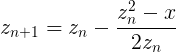# Go基础学习记录 - Go指南 - 流程控制语句:if/else

### 环境

go version go1.10.1 darwin/amd64

### if/else

Go 的 if 语句与 for 循环类似，表达式外无需小括号 ( ) ，而大括号 { } 则是必须的。

``````package main

import (
"fmt"
"math"
)

func sqrt(x float64) string {
if x < 0 {
return sqrt(-x) + "i"
}

return fmt.Sprint(math.Sqrt(x))
}

func main() {
fmt.Println(sqrt(2), sqrt(-4))
}``````

``1.4142135623730951 2i``

### if 的简短语句

``````package main

import (
"fmt"
"math"
)

func pow(x, n, lim float64) float64 {
if v := math.Pow(x, n); v < lim {
return v
}

return lim
}

func main() {
fmt.Println(
pow(3, 2, 10),
pow(3, 3, 20),
)
}``````

``9 20``

### if 和 else

``````package main

import (
"fmt"
"math"
)

func pow(x, n, lim float64) float64 {
if v := math.Pow(x, n); v < lim {
return v
} else {
fmt.Printf("%g >= %g\n", v, lim)
}

return lim
}

func main() {
fmt.Println(
pow(3, 2, 10),
pow(3, 3, 20),
)
}``````

``````27 >= 20
9 20``````

### 练习：循环与函数``````package main

import (
"fmt"
"math"
)

func Sqrt(x float64) float64 {
z := float64(1)
for n := 0; n < 10; n++ {
z = z - ((z*z - x) / (2 * z))
}

return z
}

func main() {
fmt.Println(Sqrt(2))
fmt.Println(math.Sqrt(2))
}``````

``````1.414213562373095
1.4142135623730951``````

##### 版权声明

durban创作并维护的 小绒毛的足迹博客采用创作共用保留署名-非商业-禁止演绎4.0国际许可证。

##### 版权声明

durban创作并维护的 小绒毛的足迹博客采用创作共用保留署名-非商业-禁止演绎4.0国际许可证。